# The 2nd-shortest Path

October 21, 2021

### Update Log 11.25.2021:

#### Comptability：

Known issue has been included in README.md

Recommend to compile with clang++，or Visual Studio 2019 CE.
Lab Files has been compiled and reviewed under MacOS 12.0.1，and Windows 10 + CMake 3.19 + Visual Studio Community 2019.

Set CMake minimum requirement to 3.14

#### Performance Improvement：

Using C++ 11 rvalue reference/move semantics to mitigate the copy construction overhead, 5% speed improvement under large case
Using C++11 std::future, std::async for async function calls, with 5% improvement in speed.

## Chapter 1: Introduction

Given M vertices, indexed from 1 to M, and N edges to represent a Directional Graph.

(In my implementation, both directional and undirectional graph works fine.)

To simplify the problem, the starting point is vertex 1 and the destination is vertex M.

#### Task: Find Out the Second Shortest Path

• Allow Backtracking: means each vertex can be presented in the path more than once.
• e.g. Path: 1->2->1->3 is valid
• Multiple Shortest Path: if multiple shortest path exists, the second shortest path is the one whose length is longer than those but no longer than any other path.
• e.g. The length of all possible paths are 100,100,105,110. The second shortest path length is 105.
• If two or more second shortest paths exist, any second shortest path would be accepted.

### Input Specification:

In the first line specifies the number of vertices M and number of edges N of a digraph.

Then the rest N lines, each line is consisted of a edge, given by:

• Vertex is numbered from 1 to M
• Only positive path length are considered

### Constraints:

• $1\le M\le1000$
• $1\le N\le5000$
• $1\le pathLength\le1000$

### Hint:

• If(InputSize = 1000~10^6), a tolerable upper bound of an algorithm would be $O(nlog(n))$
• If(InputSize = 10^7), a tolerable upper bound of an algorithm would be $O(n)$
• For this problem, if the input size is roughly $10^6<$M*N $<10^7$, the algorithm should be at least bounded by
• $O(MN log(MN))$ .

### Output Specification:

Output the second shortest path in the form below:

If there’s no second shortest path exist:

• Print a prompt to indicate there’s no second shortest path
• Print the shortest path in the form below:

### Sample:

• Input
• Output

In general, this is an specialization of single source K Shortest Path Problem , where K=2.

Many different methods have been proposed to solve this problem, and there are two main variants of this problem:

For DiGraph with M edges and N vertices:

This problem, is the Loopy variant, which means an optimal time complexity can be achieved at $O(n*log (n))$, which has a desirable run time with given constraints.

### Building Environment:

• MacOS 12.0.1
• VSCode
• Compiler: Clang ++ 13.0
• C++ Standard = C++ 11
• ARM64 Platform (Apple Silicon)

Using cmake for crossplatform compile

### Step2:

The Cmake instructions (CMakeLists.txt) has been provided. You can modify it yourself.

### Step3:

Open terminal/Powershell

Type in command as below

Now you’re running the program.

The program will output result carries out by each algorithm and their total runtime, in order of decreasing time complexity.

## Approach 2

Using VSCode C/C++ Extensions

## Other IDE:

Import the source files, and set the C++ standard to C++11, better compile with Visual Studio 2019, or using Clang++.

## Known Issue:

• GCC doesn’t support some C++ 11 features as of on Clang++, so if possible using clang++ instead.
• When using Visual Studio 2019 for compiling, a largest case of 5000 edges would throw error of std::vector memory limit. However, this won’t happen when compile with clang++.

### INPUT MODE:

By default we set to __FILE_INPUT_ And Looptime to 1.

USAGE ON MACROS:

• _SAMPLE_CASE_: The sample given by OJ.
• FILE_INPUT_: Get input from files.
• 1 file case is provided to test the largest possible input.
• of size M=1000, N=5000
• _USER_INPUT_: Get input from command line
• Available Cases:
• _SAMPLE_CASE_1~6
• Available Graph Type:
• class DiGraph for directional graph
• class UGraph for undirectional graph

## Chapter 2: Algorithm Specification Description

### Graph Representation:

We use the multiList representation of graph, meaning the multiList[i] indicates all the edges which starts from vertex i.

• Vertices on user interface level are numbered from 1 to n

• Vertices in the multiList are numbered from 0 to n-1

• Since variable sized array is available in C++, we don’t need linked list for the multiList implementation, using vector<vector<std::pair<int,int>>> instead.

• In the std::pair, the first one indicates the destination vertex and the second indicates the path length (or cost).

• Type of the elements in the multiList:

• We implemented two types of graph, undirected graph and directed graph.

• They differs quite little in this implementation. A undirected graph, in this case, is a specialization of the directed graph.
• An API insertEdge() is implemented for edge insertion.

### Check Connection:

Before we start to find the second shortest path, we have to firstly check if there’s a possible path from vertex 1 to vertex m. We implemented this by Breadth-First-Search.

After above process, we check if vertex m has been visited, if not, means there’s no possible path from vertex 1 to vertex m, abort.

If vertex m has been visited, means there’s at least one path from vertex 1 to vertex m.

In this case, ShortestPath from 1 to m must exist.

### Second Shortest Path:

In the Dijkstra’s algorithm, we find the shortest path by steps below:

• We keep an table, keeps track of each vertex’s shortest Path Length from Start and its direct predecessor of in shortest path.

• Each vertex is initialized as cost to infinity and predecessor to -1 as “Unmarked”

• Each time visit the Unvisited Vertex Which Has The Shortest Path Length from Start

• Compute its neighbors’ cumulative Path Length,

check if it’s shorter than the table’s records
if it's shorter, update table with the **path length and predecessor**

• After compute, Mark the Vertex as visited.

• Dijkstra’s algorithm is an Greedy Algorithm, using local minimum to get global minimum.

• It’s possible to only record the direct predecessor of each vertex and be able to get the path from the table.
• The shortest path from vertex 1 to m is the global minimum, and each edge along this path is also the global minimum from vertex 1 to that vertex.
• An table may look like this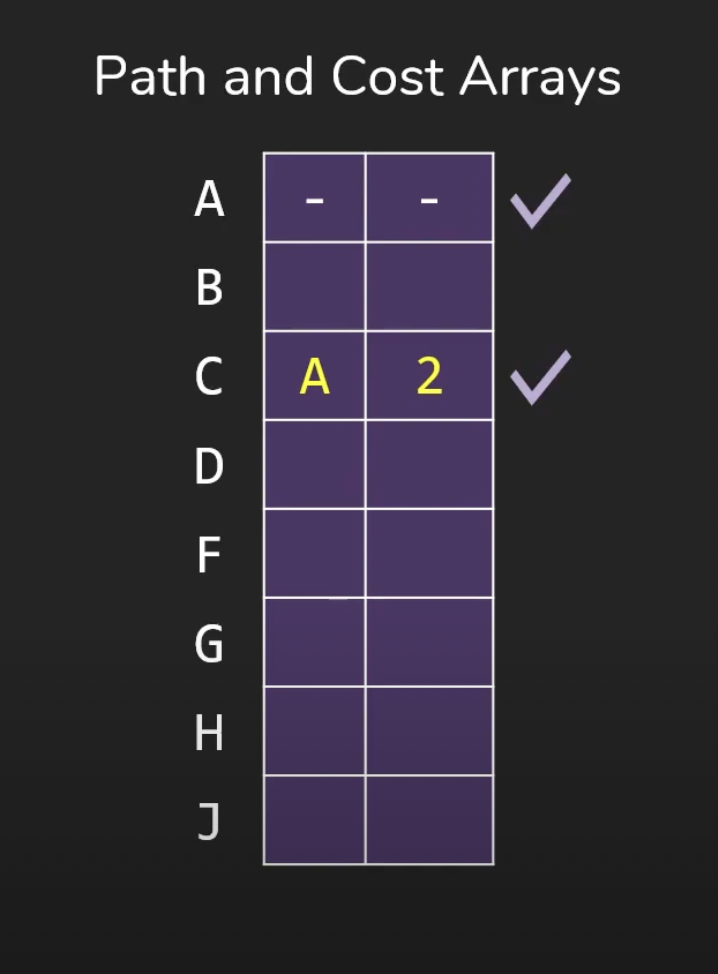Note that each step we have to find the Unvisited Vertex Which Has The Shortest Path Length from Start, If we do linear scan, the time complexity would be O(V^2). But if we keep a min heap, the time complexity would be O((V+E)log(E)).

### Make Some Extension

To find the second shortest path, we simply keep another table, record the second shortest path from vertex 1 to vertex i. And we have to keep track of all of its predecessors (in implementation, a string), instead of a single direct predecessor, since a second shortest path isn’t necessarily made up by multiple second shortest sub-paths.

We made some modification in the update part of the Dijkstra’s algorithm.

Also, to allow backtracking, each node can be pushed on to min heap multiple times. But for the second shortest path, the node in such path can appear at most twice.

This is obvious, if a path 1->3->1->2->1->4 contains a node appears more than twice (1 appears 3 times), there must exist 2 shorter paths where vertex 1 appear 1 and 2 times and reach 4, and thus it’s not a second shortest path.

The overall pseudocode looks like below:

If the second_shortest[vertexLast].cost=infinity, means there’s no second shortest path from vertex 1 to last vertex. In this case, we output the shortest path. A shortest path is ensured since we’ve done the BFS in advance.

## Chapter 3: Testing Results

#### Result: Correct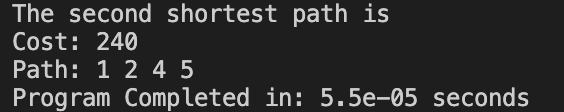#### Case 2: An Undirectional Graph#### Result: Correct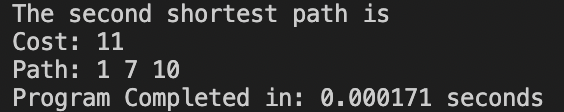#### Result: Correct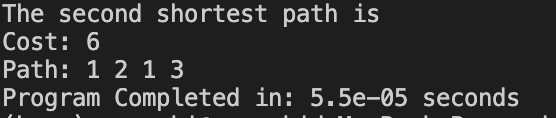#### Result: Correct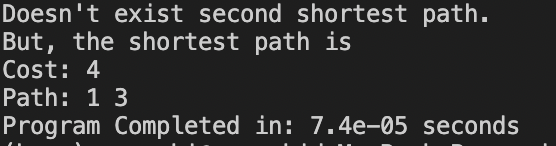#### Result: Correct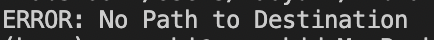#### Result: Correct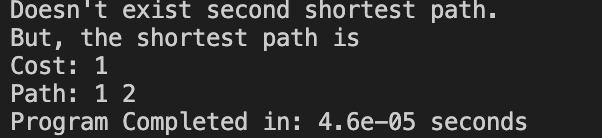#### Case 7: Maximum Case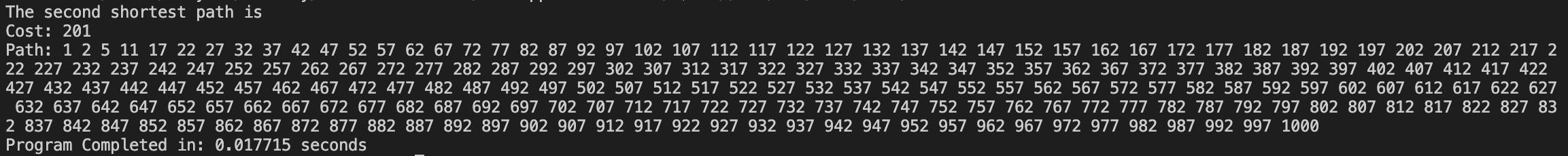### Conclusion:

• The result seems acceptable so far, need some complex test cases for validation.

## Chapter 4: Analysis and Comments

• M = number of Vertices
• N = number of Edges

### 1.Build Graph:

We keep an multiList by inserting all edges into the vector<vector<pair<int,int>>>

• Each insertion takes $O(1)$, the overall time complexity would be $O(N)$

#### Overall Time Complexity: $O(N)$

We keep an multiList, each element is consist of destination and cost, which is $O(1)$

the overall time complexity would be $O(N)$

#### Overall Space Complexity: $O(N)$

For Undirectional Graph, the only difference is that the insertion time and space taken by undirectional graph is twice as the directional one. But both are O(N) after all.

### 2.Check Connection:

1. In this step each edge is visited at most once, each node is visited at most once.
2. The enqueue and dequeue takes $O(1)$ time.

the overall time complexity would be $O(N)$

#### Space Complexity :

A queue of $O(N)$ (at most contains all edges) is needed in this step.

### 3.Second Shortest Path

• We push each node to min heap at most twice (2M)

• Each time a edge is popped

• We update table, which takes $O(1)$ time
• Each pop or push takes $O(log(M))$ time

#### Overall Time Complexity: $O(Mlog(M))$

• We keep two tables, each tables takes M elements, each elements contains a string (contains all predecessors, at most length O(N)) and a int (cumulative cost).

• Two tables occupies $O(2M*N)$ Space.
• We also keeps an heap of max size $O(N)$, each node occupies $O(1)$ Space.

### OVERALL:

#### Overall Space Complexity: $O(M*N)$

In the actual implementation of the constraint “each node is visited at most twice”, we use a slightly different approach based on the cumulative length, but they should have the same effect.

Although no hacking cases founded now, if things go wrong in some cases, the logic there needs a rewrite to be more accurate.

### Extra: K-Shortest Path

If we extend the same technique to the K shortest path, we have to keep K table, which takes up $O(KMN)$ Space.

Each node can be pushed to heap at most K times, Which takes overall $O(N + KMlog (M))$ time.